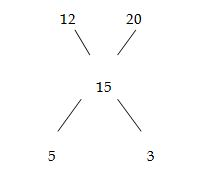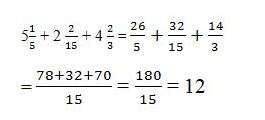# SSC CGL 2018 Practice Test Papers | Quantitative Aptitude (Day-6)

Dear Aspirants, Here we have given the Important SSC CGL Exam 2018 Practice Test Papers. Candidates those who are preparing for SSC CGL 2018 can practice these questions to get more confidence to Crack SSC CGL 2018 Examination.

[WpProQuiz 2700]

Click “Start Quiz” to attend these Questions and view Explanation

1. 25- 12 +155+ 9 = ?+160

(a) 13

(b) 14

(c) 17

(d) 16

1. Harini covered 150 km in 10 hours. She covered part of her journey by car and another part by scooter. The speed of car and scooter is 20 km/hr and 12 km/hr respectively. What is the ratio of the distances covered by car and scooter?

a) 2:3

b) 1:1

c) 4:5

d) none of the above

1. The sum of three consecutive integers is 2193. What is the value of 1/12 times of the largest number of three consecutive numbers?

(a) 63

(b) 61

(c) 51

(d) 52

1. Find the value of ?

5  1/5+ 2  2/15+ 4  2/3= ?

(a) 15

(b) 13

(c) 11

(d) 12

1. A says to B, “i am 9 times as old as you were when I was as old as you are”, if sum of their present ages 56yrs, what is A’s present age..?

a) 20

b) 18

c) 15

d) 36

6. If p = 99 then the value of p (p2 + 3p + 3)

(a) 999999

(b) 988899

(c) 989898

(d) 998889

1. In a quadrilateral ABCD, with unequal sides if the sides AC and BD intersect at right angles, then

(a) AB+BC=CD+DA

(c) AB+CD=BC+DA

(d) AB+BC=2(CD+DA)

1. A sum of Rs. 400 becomes Rs. 448 at simple interest in 2 years. In how many years will the sum of Rs. 550 amounts to Rs. 682 at the same rate?

(a) 2

(b) 3

(c) 3.5

(d) 4

1. Rs. 730 were divided among A, B, C in such a way that if A gets Rs. 3, then B gets Rs. 4 and if B gets Rs. 3.50 then C gets Rs. 3. The share of B exceeds that of C by

(a) Rs. 30

(b) Rs. 40

(c) Rs. 70

(d) Rs. 210

1. The sum of four numbers is 48. When 5 and 1 are added to the first two; and 3 & 7 are subtracted from the 3rd & 4th, the numbers will be equal. The numbers are

(a) 4, 12, 12, 20

(b) 5, 11, 13, 19

(c) 6, 10, 14, 18

(d) 9, 7, 15, 17

25- 12 +155+ 9 = ?+160

? = 17

Average speed of Harini= 150/10= 15 km/hrRatio of time taken of car to scooter is 5:3

Ratio of distance covered is 5*12 : 3*20= 1:1

Let the three numbers be x-1, x, x+1

x-1 + x + x + 1=2193

3x=2193

X=731

Largest number=731+1=732

1/12 (732) = 61Let the ages of A and B be x and 56 – x respectively.

Difference between their ages = x – (56 -x) = 2x – 56 —-> (1)

when A’s age was 56 – x
B’s Age must be (A’s age – their age difference)

B’s past age = (56-x) – (2x – 56) = 112 – 3x

it is given that A’ present age is nine times  the B’s past age:

=> x = 9(112 – 3x)
x = 36

Given P = 99 then

P (P2 + 3P + 3) = P((P + 1)2 + P + 2)

= 99 ((99 + 1)2 + 101) = 99 × (10000 + 101) = 999999

SI= PNR/100

48=400x2xr/100

r=8%

Therefore, Interest= 682-550=132

132=550 x 8 x n/100

N=3

A/B = 3/4

B /C= 3.5/3 = 7/6

and A + B + C = 730

3/4B+B+6/7B=730

B=280

C=6/7B = 6/7×280 = 240

The share of B exceeds that of C by (280 – 240) = Rs. 40

Let the four numbers be a, b, c, d respectively.

a+5=b+1,c-3=d-7

=>a=b – 4 ——— >(i)

c=d – 4 ———– >(ii)

b – 4 + b + d – 4 + d = 48

b + d = 28———- >(iii)

But we know, b + 1 = d – 7 ,

b = d – 8 ——– >(iv)

Substituting (iv) in equation  (iii)

we get  d = 18

Solving this way we get a = 6, b = 10, c = 14 and d = 18